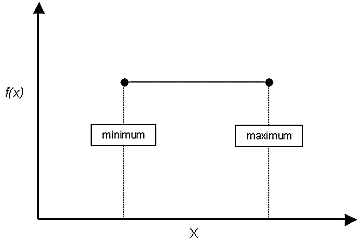The Rocscience International Conference 2021 Proceedings are now available. Read Now

Uniform Distribution

A UNIFORM distribution can be used to simulate a random variation between two values, where all values in the range are equally probable.

A UNIFORM distribution is entirely specified by the minimum and maximum values. The mean value of a UNIFORM distribution is simply the average of the minimum and maximum values, and cannot be independently specified.Uniform probability density function